# theme_test ggplot2 Theme in R (6 Examples)

This article demonstrates how to set the ggplot2 theme to the theme_test in R programming.

You’re here for the answer, so let’s get straight to the examples…

## Example Data & Packages

The following data will be used as basement for this tutorial:

```set.seed(122665)                           # Set random seed
data <- data.frame(x = rnorm(160),         # Create example data frame
y = rnorm(160),
group = LETTERS[1:4])Table 1 shows that our example data consists of three columns.

In this tutorial, we’ll also need to install and load the ggplot2 package:

```install.packages("ggplot2")                # Install & load ggplot2 package
library("ggplot2")```

## Example 1: Draw ggplot2 Scatterplot Using theme_test()

```ggplot(data,                               # theme_test scatterplot
aes(x = x,
y = y,
col = group)) +
geom_point() +
theme_test()```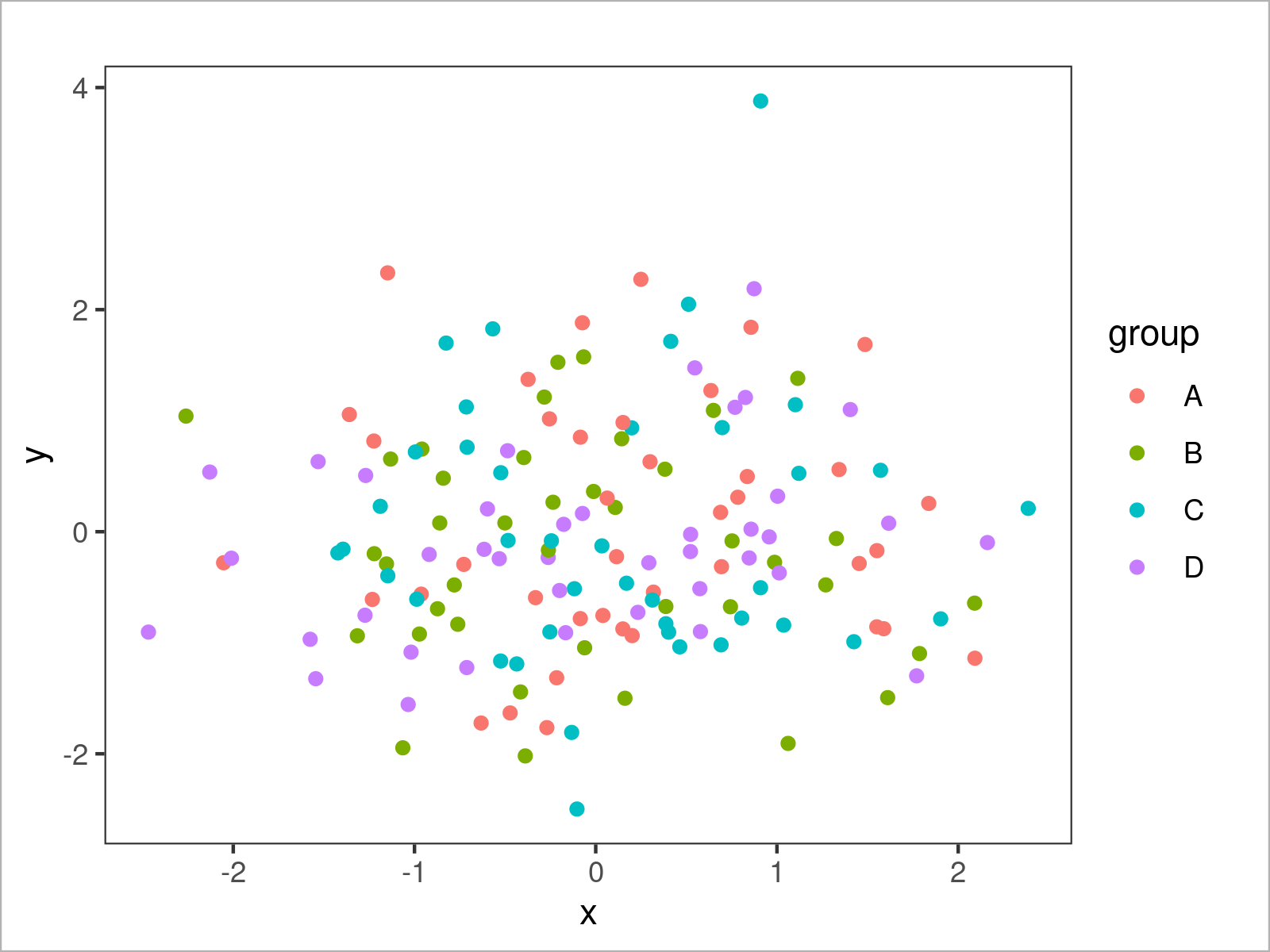## Example 2: Draw ggplot2 Density Plot Using theme_test()

```ggplot(data,                               # theme_test density plot
aes(x = x,
fill = group)) +
geom_density(alpha = 0.5) +
theme_test()```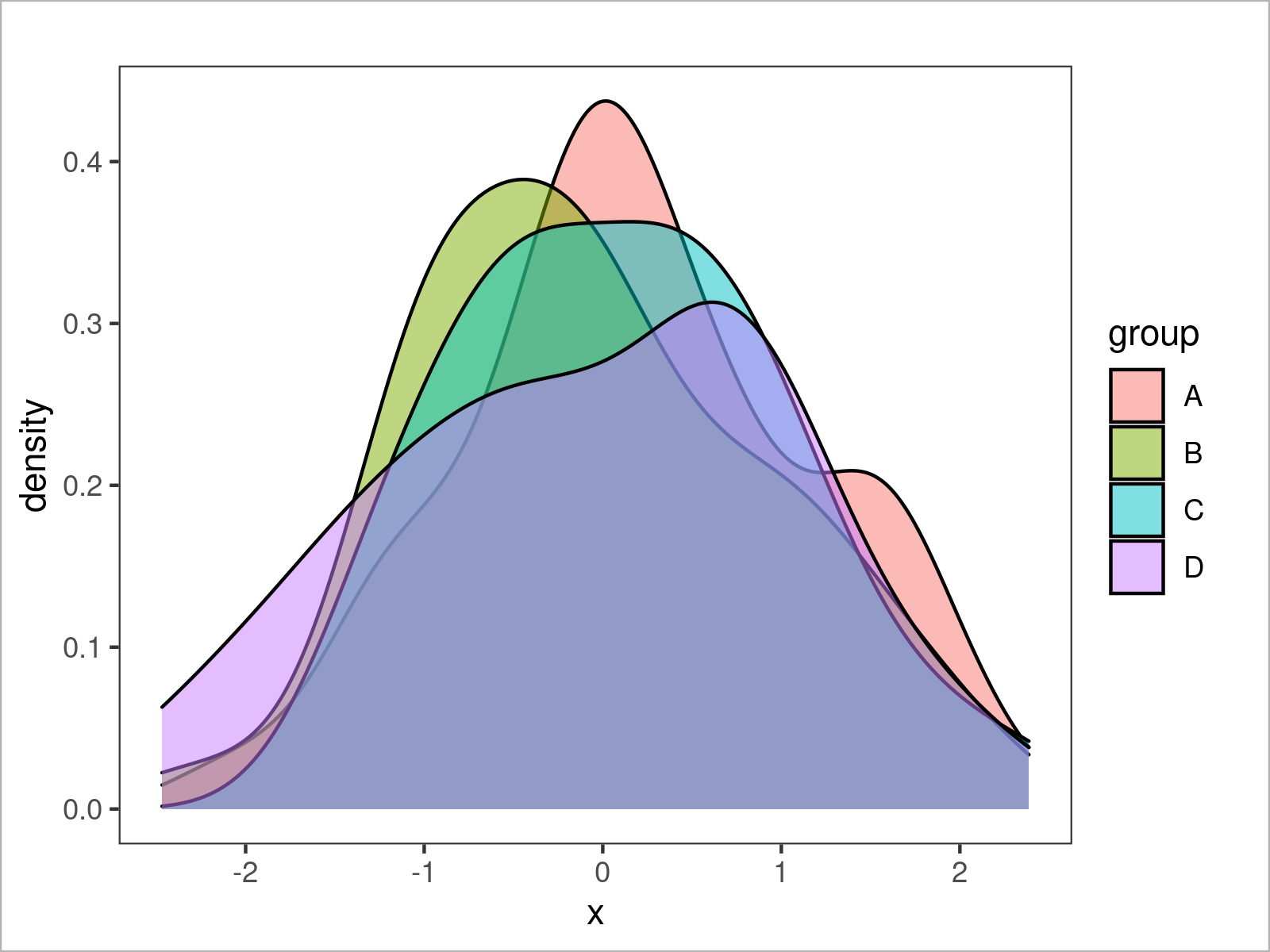## Example 3: Draw ggplot2 Histogram Using theme_test()

```ggplot(data,                               # theme_test histogram
aes(x = x,
fill = group)) +
geom_histogram(alpha = 0.5,
position = "identity",
bins = 50) +
theme_test()```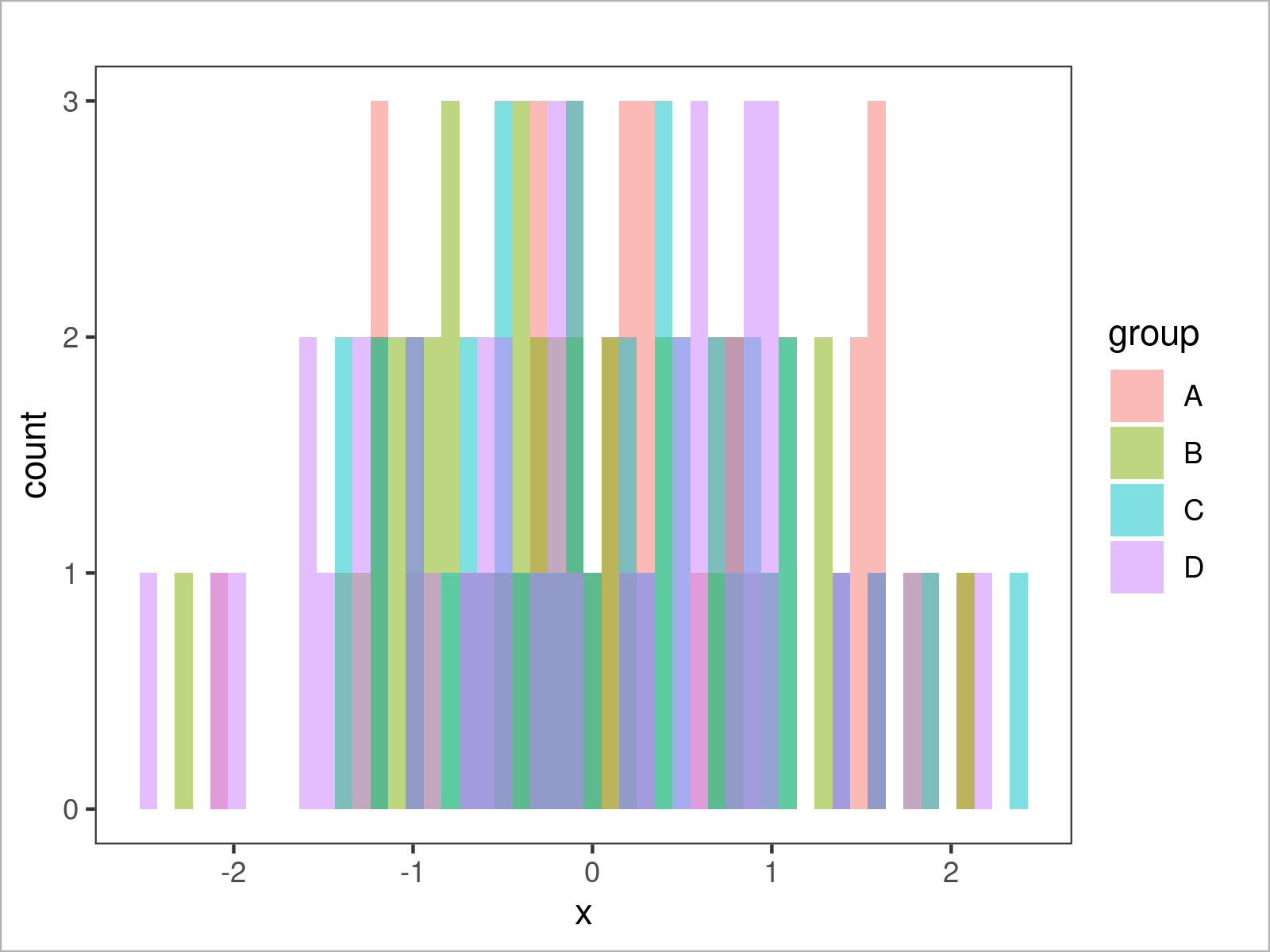## Example 4: Draw ggplot2 Barchart Using theme_test()

```ggplot(aggregate(y ~ group, data, sum),    # theme_test barplot
aes(x = group,
y = y,
fill = group)) +
geom_bar(stat = "identity") +
theme_test()```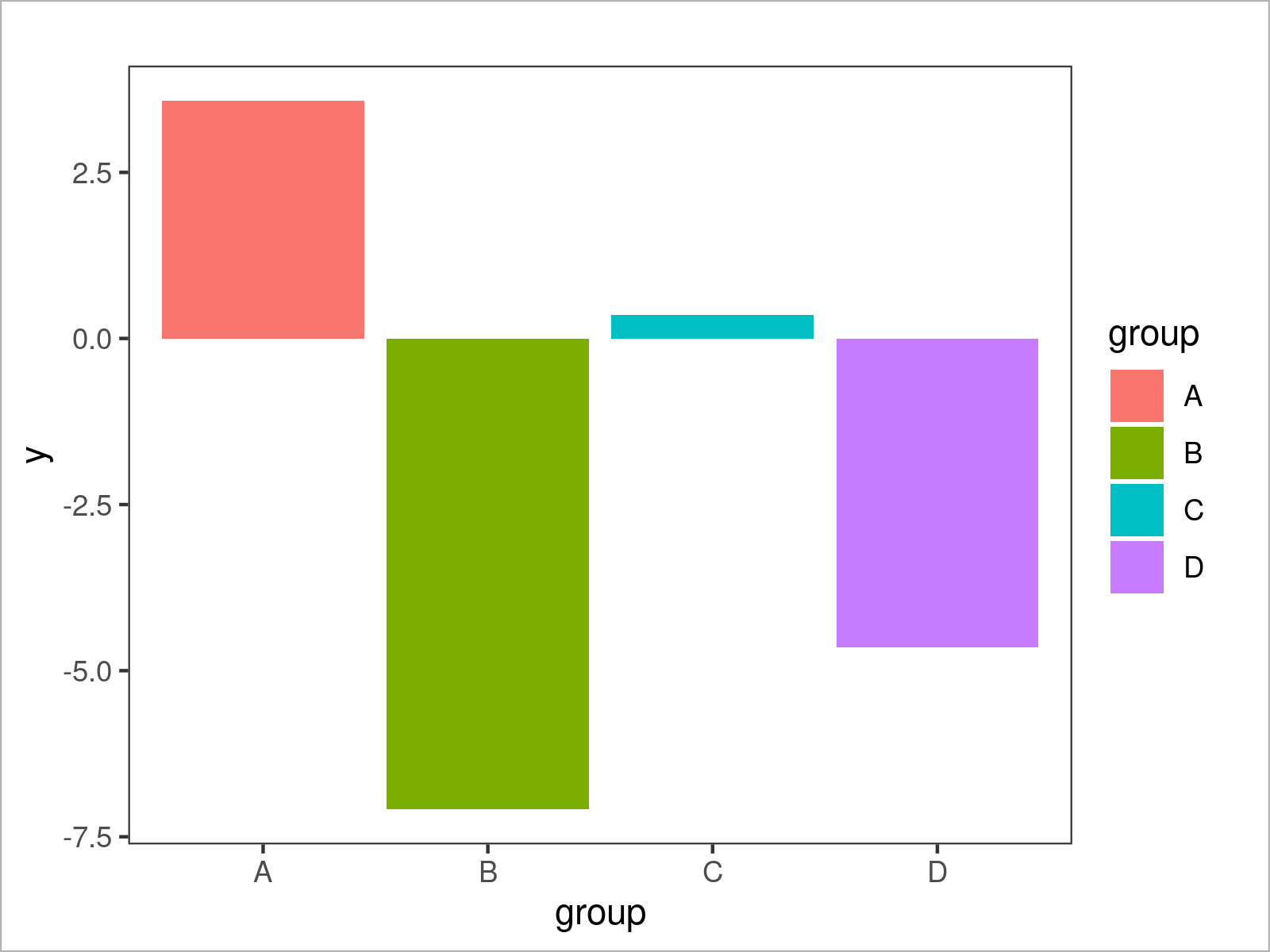## Example 5: Draw ggplot2 Boxplot Using theme_test()

```ggplot(data,                               # theme_test boxplot
aes(x = x,
fill = group)) +
geom_boxplot() +
theme_test()```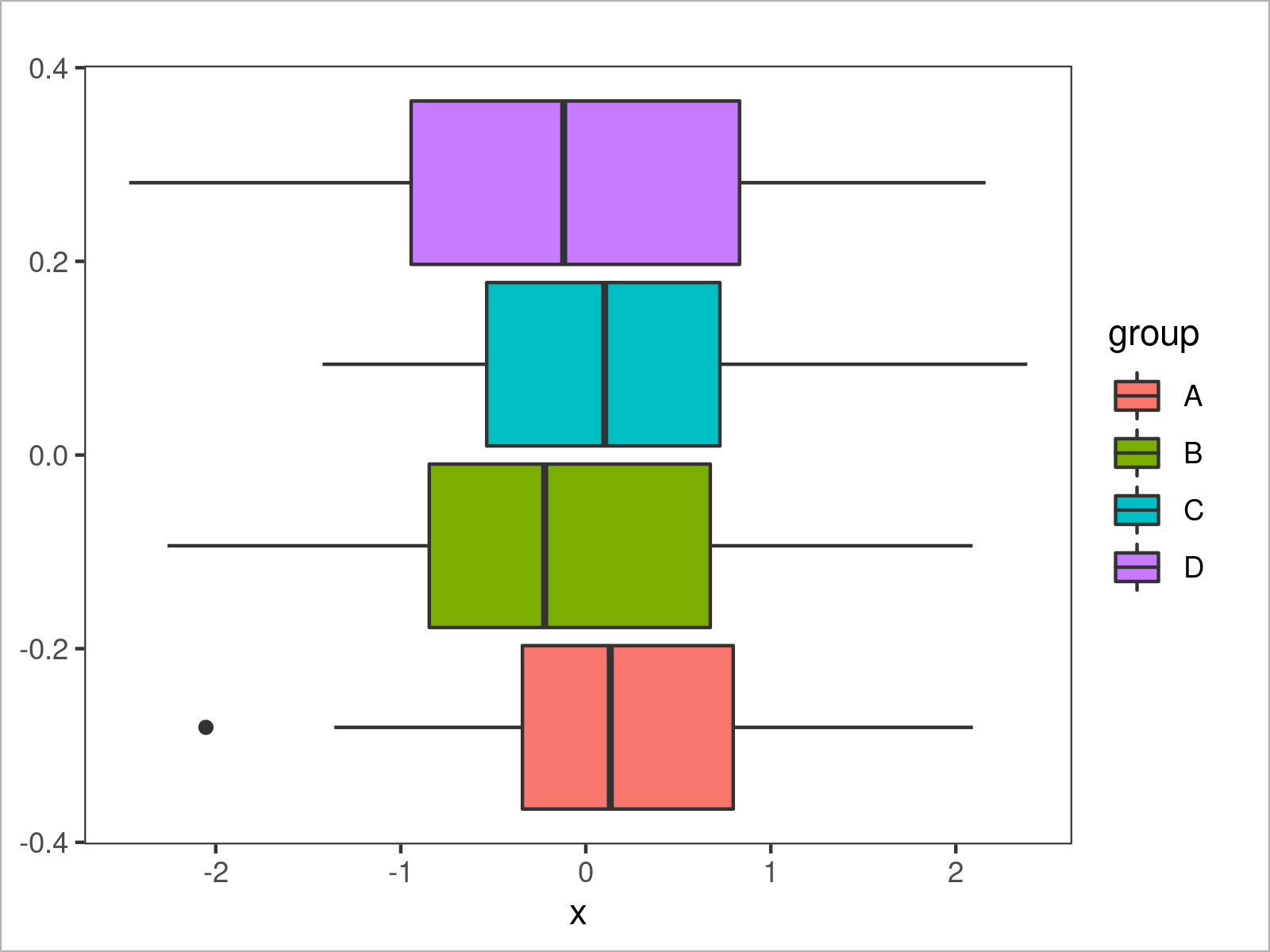## Example 6: Draw ggplot2 Line Plot Using theme_test()

```ggplot(data,                               # theme_test line plot
aes(x = x,
y = y,
col = group)) +
geom_line() +
theme_test()```## Video & Further Resources

Would you like to know more about the ggplot2 package? Then you may have a look at the following video of my YouTube channel. I explain how to use the ggplot2 package in much more detail:

Please accept YouTube cookies to play this video. By accepting you will be accessing content from YouTube, a service provided by an external third party.If you accept this notice, your choice will be saved and the page will refresh.

You can also find tutorials on how to plot different types of graphics such as xy-plots, density plots, bargraphs, boxplots, histograms, and line charts using other ggplot2 themes:

Also, you may check out the introductions to other add-on packages that provide extra themes for ggplot2 plots:

In addition, you may want to read some of the related articles on this website. You can find a selection of tutorials below.

To summarize: You have learned on this page how to apply the theme_test function to modify the design and layout of a ggplot2 plot in the R programming language. In case you have additional comments and/or questions, let me know in the comments section.

Subscribe to the Statistics Globe Newsletter